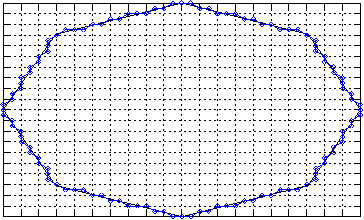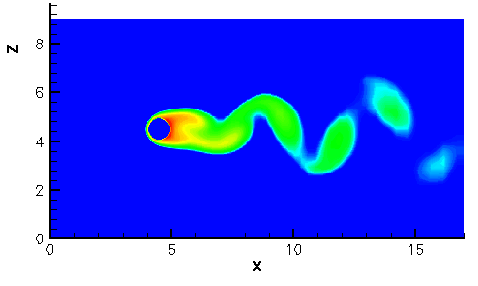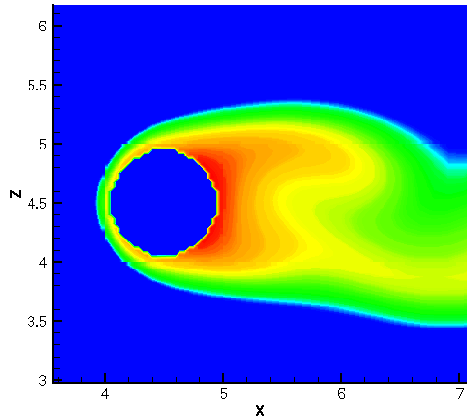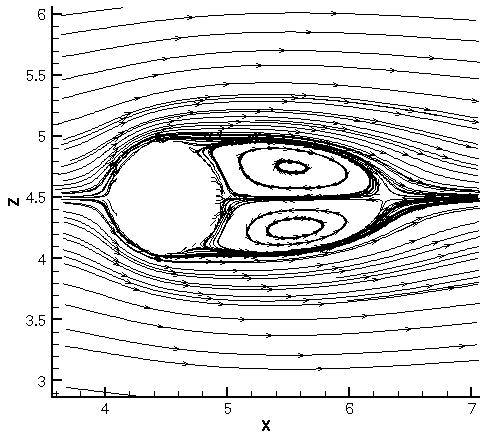Flow simulation on general bodies: Application to VKI-LES code.
Flow simulation on general bodies: Application to VKI-LES code.

Thomas Grosges
von Karman Institute for Fluid Dynamics
Chausée de Waterloo, 72
B-1640 Rhode-Saint-Genese
Belgium

Abstract

The topic of this research concerns new developments on VKI-LES code. We investigate on the three-dimensional flow around a cylinder of arbitrary cross-section, where the cylinder is of infinite extent in one direction. The toll used for the study is the EddyMD code, developed at von Karman Institute for Fluid Dynamics. It is an unsteady solver of the incompressible Navier-Stokes equations which simulate laminar and turbulent (with LES approach) flows. The computational field is treated with the Multi-Domains approach.

The main developments and evolution are consisting of including the effects due to a solid symmetrical body. In order to use the resolution methods on Cartesian grids, approximation of the body description must be realized. In the present case, the approximative description of the body is realized by a diagonal Cartesian method, based on works of Lin et al. .

The used approximation method can be used for any complex geometry. However, for complex boundaries and when a large number of grid is involved, the approximation often cannot be made manually. Therefore, an automatic method is developed to approximate the complex body in Cartesian coordinates. Figure 1 shows an example of body and its diagonal approximationFig. 1 Example of diagonal approximation on an octagonal contour.

From this description, the complex contour can now be approximated by both diagonal segments and grid lines. This method more closely approximates the actual geometry than the sawtooth method. In practice, both the original contour and the approximated one may be complex enough to have to be described by a set of discrete data points. Depending of the grid cell number, some additional neighboring points for the original contour are computed in order to have a correct diagonal approximation.

With this body description on cartesian grids, we can compute the flow field using the modified LES code, taking into account the body presence . This is done for various flow parameters. An example of such simulation result is presented at Fig. 2 for a Reynolds number, Re = 100.Fig. 2 Flow image for Re = 100.

Figures 3 and 4 show the rolls in the wake for this Re number and the average streamlines, respectively.Fig. 3 Illustration of the rolls in the wake for Re = 100.Fig. 4 Average streamlines for Re = 100.

 W. Lin, K. Carlson and C.J. Chen, Diagonal Cartesian Method for Numerical Simulation of Incompressible Flows over Complex Boundaries, Numerical Heat Transfer, Part B, 33, 181-213, 1998.
 T. Grosges, Simulation on general bodies on Cartesian grids: Application to VKI-LES code, Manual 57, von Karman Institute for Fluid Dynamics, 1-67, 2002.

oo BACK -goto- HOME oo

© Copyright 2007-2012 Thomas Grosges.